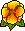## Exponential Convergence of hp-FEM for Maxwell's Equations with Weighted Regularization in Polygonal Domains

Martin Costabel, Monique Dauge, Christoph SchwabThe time-harmonic Maxwell equations do not have an elliptic nature by themselves. Their regularization by a divergence term is a standard tool to obtain equivalent elliptic problems. Nodal finite element discretizations of Maxwell's equations obtained from such a regularization converge to wrong solutions in any non-convex polygon.

Modification of the regularization term consisting in the introduction of a weight restores the convergence of nodal FEM, providing optimal convergence rates for the h Version of Finite Elements, see Paper.

We prove exponential convergence of hp FEM for the weighted regularization of Maxwell's equations in plane polygonal domains provided the hp-FE spaces satisfy a series of axioms. We verify these axioms for several specific families of hp finite element spaces.16 June 2004.

SAM Report, ETH Zürich, N. 2004-05 .

M3AS: Mathematical Models and Methods in Applied Sciences, Vol. 15, n° 4, 2005, 575-622.Pdf file (380 k)